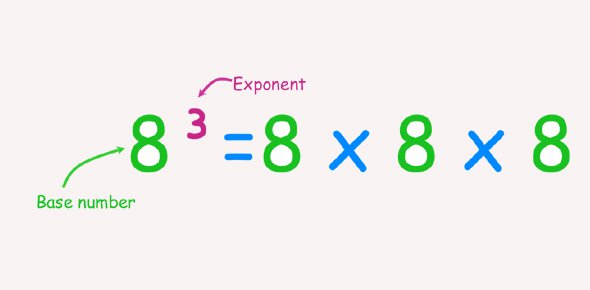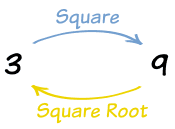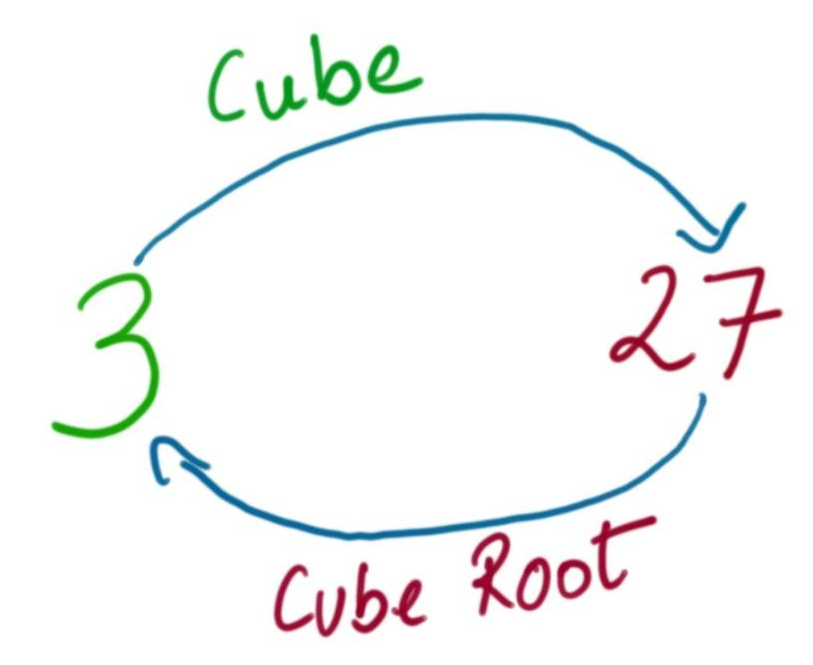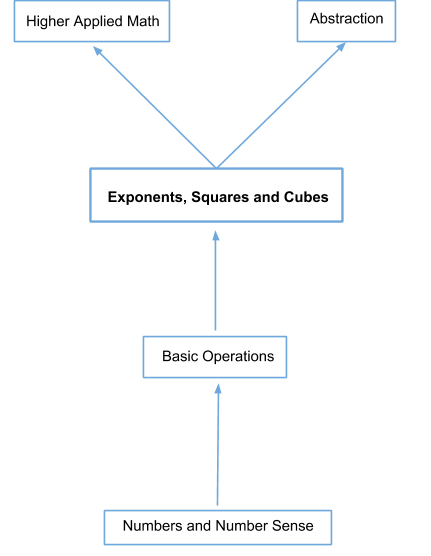In the verge of coronavirus pandemic, we are providing FREE access to our entire Online Curriculum to ensure Learning Doesn't STOP!

# Exponents, Squares and Cubes

Go back to  'Maths'

## Introduction to Exponents, Squares and Cubes

You can write 2 x 2 = 4 without much difficulty, or even 3 x 3 x 3 = 27, however, what do you do when you need to represent 2 multiplied 10 times to itself? Or when abstraction becomes involved? Mathematicians have already faced and addressed this particular hurdle and that brings us to the topics of exponents, squares and cubes.

## The Big Idea: What are Exponents, Squares and Cubes?

Exponents, squares and cubes are a mathematical tool to express and calculate higher arithmetic calculations and also, find significant application in basic, intermediate and advanced abstraction.

An Exponent is a way to represent repeated multiplication of a number/variable with itself.Squares and Cubes are limiting cases of exponents where the exponent component is always 2 or 3 respectively.

### Square Roots

Just like how squares represent a number or variable multiplied by itself twice, a square root stands for the number that needs to be multiplied twice to get that particular number. Essentially it is the inverse operation of a square.In an equation, a square root is represented by the following symbol;Numbers which yield whole numbers when their square roots are calculated are called Perfect Squares.

### Cube Roots

Similar to square roots, cube roots are the inverse operations of cubes. So, if a cube represents a number multiplied by itself thrice, the cube root of a number represents the number that is multiplied 3 times to give the original number.The symbol that represents a cube root in an expression is as follows;A number that yields a whole number when its cube root is calculated is called a Perfect Cube.

## How do I understand

### The Foundational Nature of Exponents, Squares and Cubes## Sub Topics

Here are a few links that will take you through the journey that every Cuemath students undertakes in the pursuit of understanding Exponents, Squares and Cubes along with practice worksheets:

## How to Teach Your Child Exponents, Squares and Cubes

Exponents, squares and cubes further advances the applications of arithmetic and finds widespread use in abstraction as well. That’s why it is critical that children understand the concepts at play that govern these operations. Here are a few methods that will ensure that your child masters these operations:

Chunking: An age-old practice that has survived the test of time due to its effectiveness in helping children grasp new concepts by platforming off old ones. So, in your child’s study routine for exponents, squares and cubes, dedicate a certain amount of time for them to review older concepts from multiplication, division and basic algebra.

Flash Cards: These are an excellent tool to allow your child to have all the important concepts of a topic at their fingertips. Instruct them to practice answering both conceptual and arithmetic problems using timed flash cards to not only juggle their concepts, but also aid in their mental math abilities.